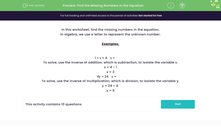# Find the Missing Numbers in the Equation

In this worksheet, students develop their algebra skills by finding the missing numbers in the given equations.Key stage:  KS 2

Curriculum topic:   Algebra

Curriculum subtopic:   Express Problems Algebraically

Popular topics:   Algebra worksheets

Difficulty level:#### Worksheet Overview

In this worksheet, find the missing numbers in the equation.

In algebra, we use a letter to represent the unknown number.

Examples:

1 + x = 4.   x =

To solve, use the inverse of addition, which is subtraction, to isolate the variable x.

x = 4 - 1

x = 3

4y = 24.   y =

To solve, use the inverse of multiplication, which is division, to isolate the variable y.

y = 24 ÷ 4

y = 6

### What is EdPlace?

We're your National Curriculum aligned online education content provider helping each child succeed in English, maths and science from year 1 to GCSE. With an EdPlace account you’ll be able to track and measure progress, helping each child achieve their best. We build confidence and attainment by personalising each child’s learning at a level that suits them.

Get started#### Popular Maths topics

••••